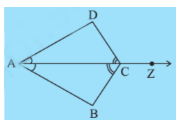## Filters

Sort by :
Clear All
Q

10.  Explain, why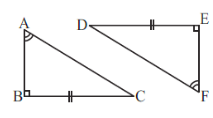Comparing both triangles, we have, So By RHS congruency criterion,  .

8. Draw a rough sketch of two triangles such that they have five pairs of congruent parts but still the triangles are not congruent.

Five pairs of congruent parts can be three pairs of sides and two pairs of angles. In that case, the SAS or ASA criterion would prove them to be congruent. Hence, such a figure is not possible.

7.  In a squared sheet, draw two triangles of equal areas such that
(i) the triangles are congruent.
(ii) the triangles are not congruent.

What can you say about their perimeters?

When two triangles are congruent, the corresponding parts are exactly identical so they have the same area and perimeter. While the triangles are not congruent but have the same area, then the perimeter of both triangles are not equal.

9. If and  are to be congruent, name one additional pair of corresponding parts. What criterion did you use?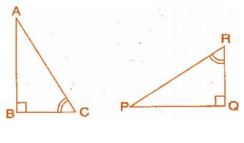Given  One additional pair which is not given in the figure is  We used the ASA Criterion as the two corresponding angles are given and we figured out the side by congruency.

6. Complete the congruence statement: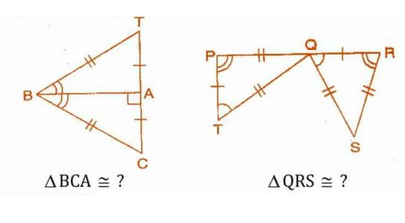Comparing from the figure, we get, So By SSS Congruency Rule,    Also, Comparing from the figure, we get, So By SSS Congruency Rule,   .

5. In the figure, the two triangles are congruent. The corresponding parts are marked. We can write ?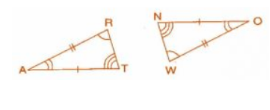Comparing from the figure. By SAS Congruency criterion, we can say that  ]

4. In , , and .

In ,  , and . A student says that by AAA congruence criterion. Is he justified? Why or why not?

No, because it is not necessary that two triangles will be congruent if their all three corresponding angles are equal. in this case, the triangles might be zoomed copy of one another.

2.(c) You want to show that

(c) If it is given that  and you are to use ASA criterion, you need to have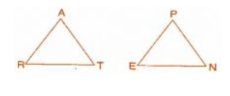Given,  also,  Now, As we know in the ASA criterion of proving congruency, the one and side two angles are equal to their corresponding parts. So,

2.(b)  You want to show that

(b) If it is given that  and you are to use SAS criterion, you need to have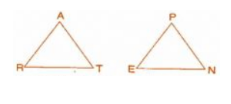As we know in SAS  criterion the two sides and one angle are identical to their corresponding parts of another triangle. So to prove congruency we need to prove that,

2.(a)  You want to show that

(a) If you have to use  criterion, then you need to show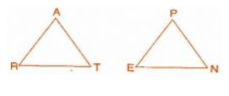As we know that in the criterion of proving congruent, all three corresponding sides are equal to another. So to prove the congruency, we kneed to know the following things:

3. You have to show that . In the following proof, supply the missing reasons.

 Steps Reasons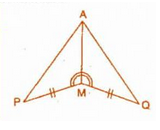Steps     Reasons    Given in the question    Given in the question.    the side which is common in both triangle     By SAS Congruence Rule

1.(c)  Which congruence criterion do you use in the following?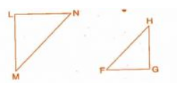Since we are comparing two angles and one side, ASA(Angle, Side, Angle) congruency criterion is used to prove the congruency.

1.(b)   Which congruence criterion do you use in the following?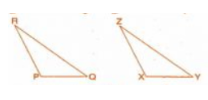Since we are comparing two sides and one angle of the two triangles, the SAS (sie, angle, side) congruent criterion is used to prove them congruent.

1.(a)   Which congruence criterion do you use in the following?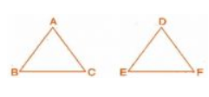Since we are comparing all the sides of two triangles, The SSS (side, side, side) Congruent criterion is used.

1.(d) Which congruence criterion do you use in the following?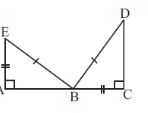Since we are comparing two sides and one angle of the two triangles, the SSA (Side, Side, Angle) congruent criterion is used to prove the congruency.

4. ABC is an isosceles triangle with and is one of its altitudes (Fig 7.34).

(i) State the three pairs of equal parts in and .
(ii) Is ? Why or why not?
(iii) Is ? Why or why not?
(iv) Is ? Why or why not?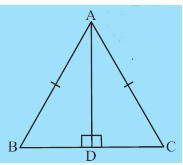i) Given in and .    ( Common side)    ii) So, by RHS Rule of congruency, we conclude   iii) Since both triangles are congruent all the corresponding parts will be equal. So,   iv) Since both triangles are congruent all the corresponding parts will be equal. So, .

3. In Fig 7.33, and are altitudes of  such that .

(i) State the three pairs of equal parts in and .
(ii) Is  ? Why or why not?
(iii) Is  ? Why or why not?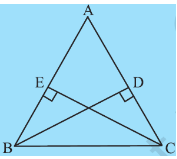i) Given,  in and .     ii) So, By RHS Rule of congruency, we conclude:   iii) Since both the triangle are congruent, all parts of one triangle are equal to their corresponding part from another triangle. So. .

2. It is to be established by RHS congruence rule that  . What additional information is needed, if it is given that

and

To prove congruency by RHS (Right angle, Hypotenuse, Side ) rule, we need hypotenuse and side equal to the corresponding hypotenus and side of different angle. So Given   ( Right angle )    ( Side ) So the third information we need is the equality of  Hypotenuse of both triangles. i.e. Hence, if this information is given then we can say, .

1.  In Fig 7.32, measures of some parts of triangles are given.By applying RHS congruence rule, state which pairs of triangles are congruent. In case of congruent triangles, write the result in symbolic form.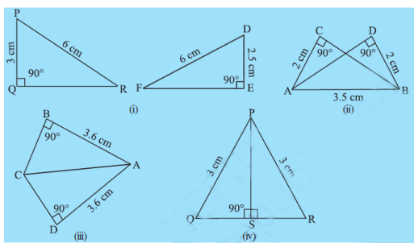i) In  and  Hence they are not congruent. ii)  In  and   ( same side ) So, by RHS congruency rule, iii) In  and   ( same side ) So, by RHS congruency rule, iv)  In  and   ( same side ) So, by RHS congruency rule,

5. In Fig 7.28, ray AZ bisects  as well as .

(i) State the three pairs of equal parts in triangles  and .
(ii) Is  Give reasons.